Next: The relativistic law for velocity addition Up: Paradoxes of lengths shortening Previous: Problems on thin rods   Contents

## Some remarks on lengths shortening

We shall additionally consider now the relativistic effect of contraction of distances (the paradox of pedestrians). We shall "agree in advance" about the following mental experiment (Fig. 1.20).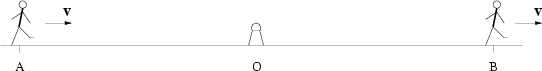Let a beacon, disposed at the middle of a segment, to send a signal toward its ends. Let segment's length be one million light years. At the time of arrival of a flash two pedestrians at segment's ends begin to walk at equal velocity toward the same preselected side, along the straight line containing the given segment, and they will be walking for several seconds. The moving segment (a system of two pedestrians) should be contracted relative to the ends of a motionless segment by some hundreds kilometers. However, none of pedestrians will "fly away" for hundreds kilometers during these seconds. The moving segment could not also be torn off at the middle, because the Lorentz transformation laws are continuous. So, where has this segment been contracted in such a case? And how this can be detected?

For "justifying" the relativistic contraction of lengths Fock  discusses as follows. In the motionless coordinate system the lengths (factually fixed by tips of a segment) can be measured non-simultaneously, but in the moving system they must be measured simultaneously. From the invariance of the interval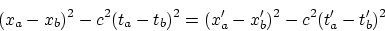at the choice of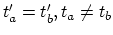we obtain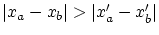. But in such a case, why we can not choose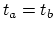arbitrarily in order to obtain the objective length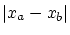in a unique manner? The existence of the process of measuring the length (the tips of a segment), which is independent of time and of the concept of simultaneity for the intrinsic frame of reference, proves a full independence of time and spatial characteristics in this system. But why for the other, moving system must arise any new additional link between the coordinates and time except the kinematic concept of velocity?

Wrong is Mandelshtam's  judgement, that there is no "real length", and his example with the angular measure of an object. The angular measure of an object depends not only on object's size, but also on the distance to it, that is, on two parameters. Therefore, this measure can be made unique only if one parameter - the distance to an object - is fixed. Incorrect is also Mandelshtam's statement, that in any method of measuring the lengths the rods moving in different manner have different lengths. For example, possible is the procedure of measurement (direct comparison) of the rods previously turned perpendicular to the relative motion of the rods. Then the rods can be turned in arbitrary manner. They could even be slowly rotating in order to occur to be perpendicular to the motion at the time of coincidence. In such a case this method is completely independent on the relative motion even in SRT.

Some relativists believe that there is no length contraction at all - only the turning exists, for example, for a cube (i.e. they cannot unambiguously agree even between each other). The absence of real turning of a cube (or the fact that this effect is only apparent) can easily be proved, if the cube will fly being pressed against a ceiling. Generally speaking, the distance to objects, their visible velocity and size can be determined, even with the help of the light, by several techniques which are "self-consistent" by themselves. For example, even for a single observer: from the angular size, from illumination, from the Doppler effect. But the obtaining of different values for the same physical quantity does not cancel at all the only true objective characteristics of a body and its motion (under which the instruments are calibrated).

The SRT tries to "purchase" the consistency of its determination of lengths by refusal from the objectivity of some other physical quantities. However, this trick won't "work" with respect to the time - it is irreversible. Note some strange thing: in the sense of reversibility (in transition from one inertial frame of reference to the other and back!) the linear Lorentz transformation are fully equivalent both for coordinates and for the time (they are reversible). It seems strange, then, that a difference between bodies' lengths vanishes with return at initial place (for twins, for example), but the disparity remains in the time elapsed.Next: The relativistic law for velocity addition Up: Paradoxes of lengths shortening Previous: Problems on thin rods   Contents
Sergey N. Arteha